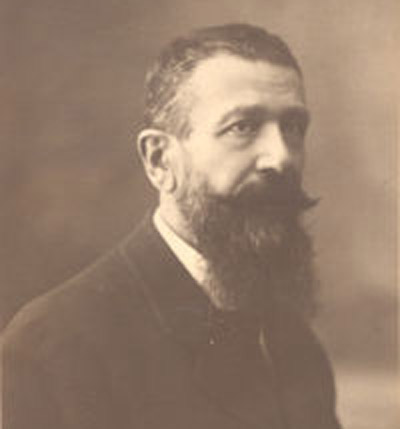A# DarlingCesare Burali-Forti (1861–1931).

The Burali-Forti paradox is an argument which shows that the collection of ordinal numbers (numbers that give the position of objects) do not, unlike the natural numbers, form a set. Each ordinal number can be defined as the set of all its predecessors. Thus,

0 is defined as {}, the empty set
1 is defined as {0} which can be written as {{}}
2 is defined as {0, 1} which can be written as {{}, {{}}}
3 is defined as {0, 1, 2} which can be written as {{}, {{}}, {{}, {{}}}}
...
in general, n is defined as {0, 1, 2, ... n - 1}

If the ordinal numbers formed a set, this set would then be an ordinal number greater than any number in the set! This contradicts the assertion that the set contains all ordinal numbers. Although the ordinal numbers don't form a set, they can be regarded as a collection called a class.